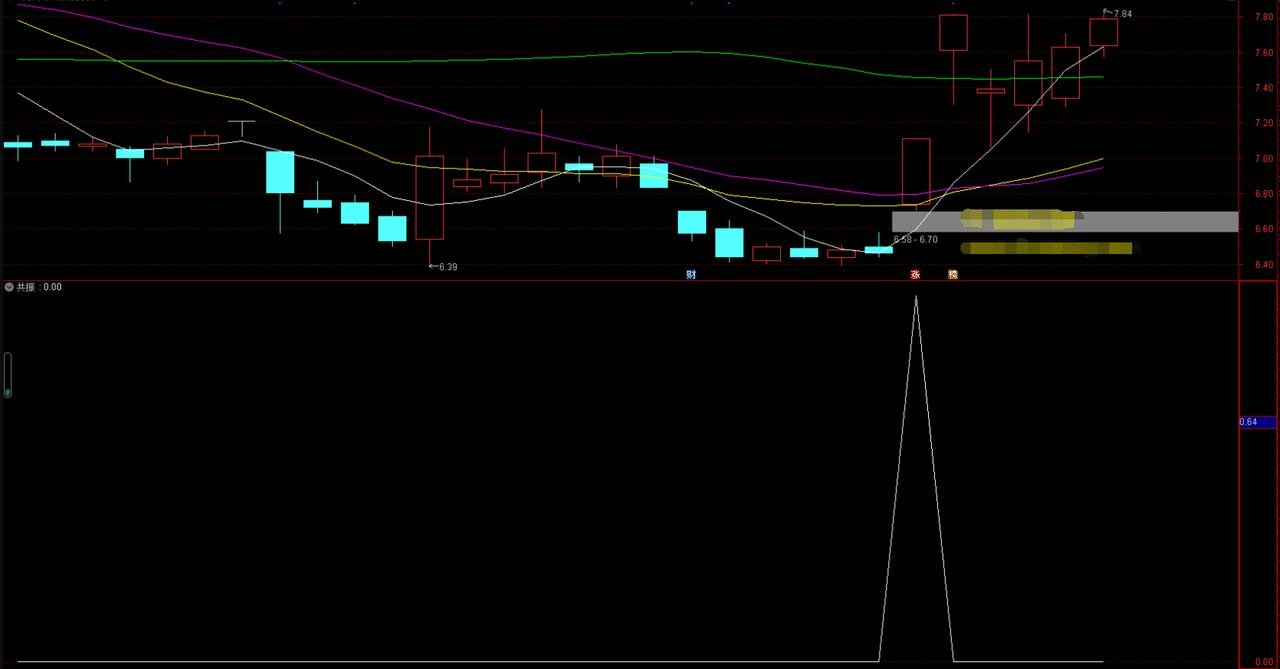# 均线共振副图源码分享，以突破程序化交易模型为主

均线共振副图源码分享，以突破程序化交易模型为主

源码：

RSV:=(CLOSE-LLV(LOW,9))/(HHV(HIGH,9)-LLV(LOW,9))*100;

K:=SMA(RSV,3,1);

D:=SMA(K,3,1);

J:=3*K-2*D;

XG:=COUNT(CROSS(K,D),2)=1;

LC:=REF(CLOSE,1);

RSI1:=SMA(MAX(CLOSE-LC,0),6,1)/SMA(ABS(CLOSE-LC),6,1)*100;

RSI2:=SMA(MAX(CLOSE-LC,0),12,1)/SMA(ABS(CLOSE-LC),12,1)*100;

RSI3:=SMA(MAX(CLOSE-LC,0),24,1)/SMA(ABS(CLOSE-LC),24,1)*100;

XG1:=COUNT(CROSS(RSI1,RSI2),2)=1;

DIFF:=EMA(CLOSE,12)-EMA(CLOSE,26);

DEA:=EMA(DIFF,9);

MACD:=2*(DIFF-DEA);

XG2:=COUNT(CROSS(DIFF,DEA),2)=1;

CROSS(C,MA(C,20)) AND XG AND XG1 AND XG2;

具体可供大家自行测试，注：不会程序化的，不要贸然尝试，在RB这开户的不少粉丝，好奇程序化，都被程序化坑亏了不少资金~【版权声明】
1.本站文章禁止复制转载等一切途径，请广大粉丝注意，如果您喜欢RB，可以收藏本站，随时访问；
2.如标题下方的来源标识为“互联网”，则来源于网络其他平台，如对原著有冒犯之处可以联系RB，予以删除文章处理；
3.RB一切只为帮助广大交易者，所以大家可以通过心得文章多参考和借鉴，但是在实战交易中需要三思而后行，毕竟交易有风险，投资需谨慎；• 全部评论（0
还没有评论，快来抢沙发吧！